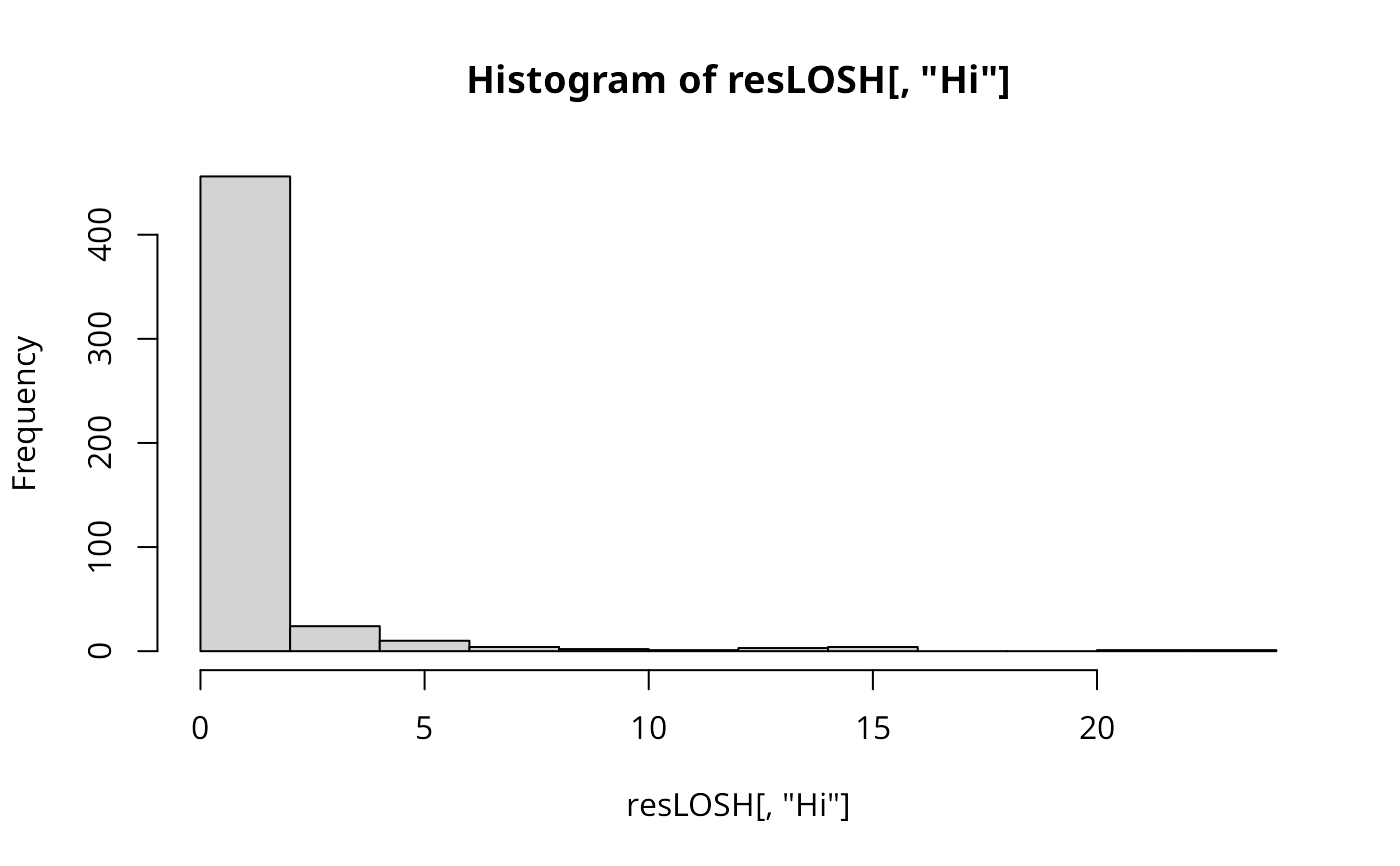The function implements the chi-square based test statistic for local spatial heteroscedasticity (LOSH) as proposed by Ord & Getis (2012).

LOSH.cs(x, listw, zero.policy = attr(listw, "zero.policy"), na.action = na.fail,
p.adjust.method = "none", spChk = NULL)

## Arguments

x

a numeric vector of the same length as the neighbours list in listw

listw

a listw object created for example by nb2listw

zero.policy

default attr(listw, "zero.policy") as set when listw was created, if attribute not set, use global option value; if TRUE assign zero to the lagged value of zones without neighbours, if FALSE assign NA

na.action

a function (default na.fail), can also be na.omit or na.exclude - in these cases the weights list will be subsetted to remove NAs in the data. It may be necessary to set zero.policy to TRUE because this subsetting may create no-neighbour observations. Note that only weights lists created without using the glist argument to nb2listw may be subsetted. If na.pass is used, zero is substituted for NA values in calculating the spatial lag. (Note that na.exclude will only work properly starting from R 1.9.0, na.omit and na.exclude assign the wrong classes in 1.8.*)

a character string specifying the probability value adjustment for multiple tests, default "none"; see p.adjustSP. Note that the number of multiple tests for each region is only taken as the number of neighbours + 1 for each region, rather than the total number of regions.

spChk

should the data vector names be checked against the spatial objects for identity integrity, TRUE, or FALSE, default NULL to use get.spChkOption()

## Details

The test uses a = 2 (see LOSH) because chi-square based inference is not applicable with other exponents. The function makes use of LOSH in its calculations.

## Value

Hi

LOSH statistic

E.Hi

expectation of LOSH

Var.Hi

variance of LOSH

Z.Hi

the approximately chi-square distributed test statistics

x_bar_i

local spatially weighted mean values

ei

residuals about local spatially weighted mean values

Pr()

p-values for Hi obtained from a non-central Chi-square distribution with $$2/Var.Hi$$ degrees of freedom

## Author

René Westerholt rene.westerholt@tu-dortmund.de

LOSH, LOSH.mc
    data(boston, package="spData")